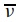# Infrared Spectroscopy

| Home | | Organic Chemistry |

## Chapter: Organic Chemistry : Structure Determination of Organic Compounds

Infrared spectroscopy is a very useful spectroscopic tool for determining the presence of functional groups and bonding sequences in a compound by the absorption of light in the IR region of the electromagnetic spectrum.

INFRARED SPECTROSCOPY

Infrared spectroscopy is a very useful spectroscopic tool for determining the presence of functional groups and bonding sequences in a compound by the absorption of light in the IR region of the electromagnetic spectrum. The IR region comprises light with wavelengths from about 1 × 104 to 8 × 107 m (100 – 0.8 µm) and lies between the microwave region and the visible region of the spectrum. The wavelengths of greatest interest to organic chemists range from 2.5 to 15 µm, the so-called mid-IR region, because the greatest amount of structural information can be obtained by spectroscopy in this spectral region.

Infrared radiation, like any electromagnetic radiation, is characterized by prop-erties of frequency (ν) and wavelength (λ) that are related by the speed of light c:

c = λv

or

v = c/λ

To scale frequency to a more convenient range, IR spectroscopists have defined a frequency unit called wave numbergiven by= 1/λ, where λ is the wave-length in centimeters. The units ofare reciprocal centimeters (cm−1). The wave number is the number of vibrations which occur over a 1-cm distance. Thus the higher the wave number, the more vibrations occur in a 1-cm distance and thus the higher the frequency. Normally IR spectra are recorded between 4000 and 650 cm−1 (2.5 and 15 µm).

The energy of IR radiation is given by E = and thus

E = h/cThe energy of the IR light absorbed by molecules during IR spectroscopy is typically in the range of 9 – 2.5 kcal/mol (for 4000 – 650-cm1 light). This amount of energy is not enough to break bonds in molecules, but it is enough to cause transitions in vibrational modes in the molecule. Thus IR spectroscopy is best described as nondestructive, vibrational spectroscopy.

As was discussed in the chapter on chemical bonding, a molecule can simplis-tically be thought of as a collection of bonds which hold the nuclei together in certain spatial relationships so that the lowest possible energy for the molecule is achieved. Deformations from these optimal angles and distances correspond to bond stretchings and bendings and these are examples of vibrational motion in the molecule. Since vibrational motions, which include both bond-stretching and bond-bending modes of vibration, are quantized, each vibrational mode in the molecule absorbs energy at a particular frequency (which happens to fall in the IR region). This provides the basis for IR spectroscopy. By determining which frequencies of IR radiation are absorbed by a molecule, it is possible to conclude what types of vibrational modes are absorbing energy in the molecule and consequently what atoms and bonds (functional groups) are present in the molecule which give rise to these vibrational modes.

A molecule which contains n atoms will have 3n 6 fundamental modes of molecular vibration. These 3n 6 fundamental vibrational motions can be divided into two types— stretching modes, of which there are n 1, and bend-ing modes, of which there are 2n 5. Stretching vibrations are those in which the internuclear distances between bonded elements change. Bending vibrations are those in which bond angles change. In general, it takes more energy to stretch a bond than to deform bond angles. Therefore absorption frequencies which corre-spond to bond-stretching modes are often higher (higher energy) than absorption frequencies which correspond to bending modes (lower energy). Stretching fre-quencies are normally found in the higher frequency portion of the spectrum (4000 – 1200 cm1) while bending frequencies are found in the lower frequency region (1200 – 600 cm1). Furthermore, it is the stretching frequencies which give the most clearcut structural information about a compound.